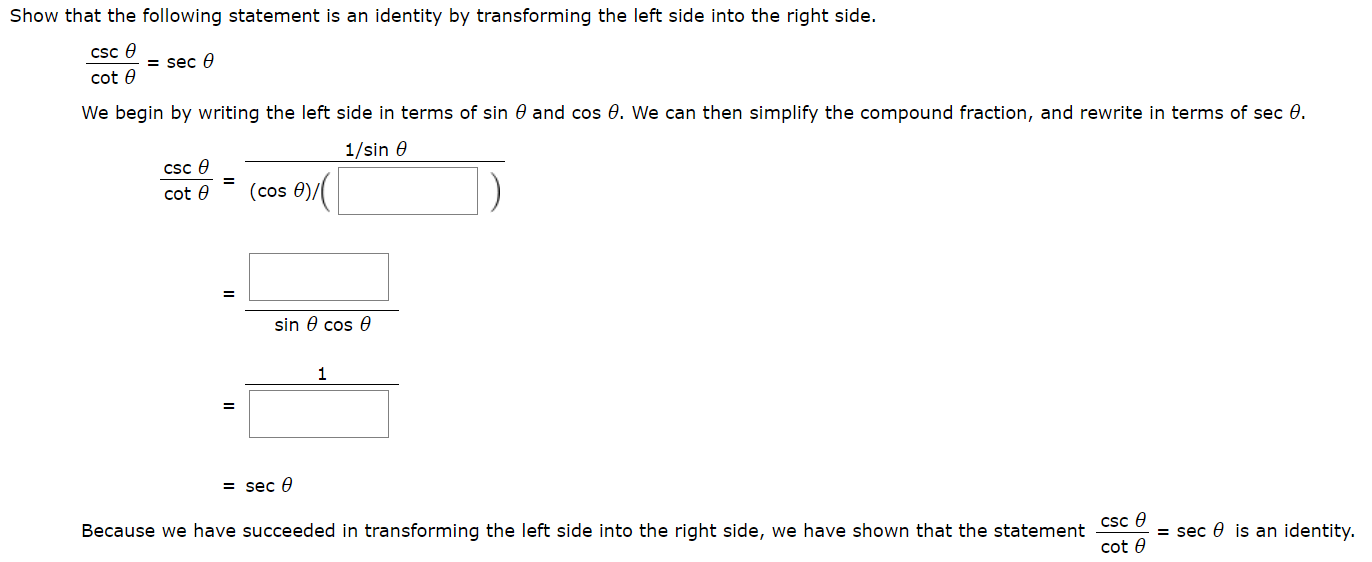# Show that the following statement is an identity by transforming the left side into the right side.csc e= seccot eWe begin by writing the left side in terms of sin 0 and cos 0. We can then simplify the compound fraction, and rewrite in terms of sec 0.1/sin ecSc e(cos 0),cot esin 0 cos e1= sec eBecause we have succeeded in transforming the left side into the right side, we have shown that the statement Csc 0= sec 0 is an identity.cot e

Question
1 views

practice question, I attached a picturehelp_outlineImage TranscriptioncloseShow that the following statement is an identity by transforming the left side into the right side. csc e = sec cot e We begin by writing the left side in terms of sin 0 and cos 0. We can then simplify the compound fraction, and rewrite in terms of sec 0. 1/sin e cSc e (cos 0), cot e sin 0 cos e 1 = sec e Because we have succeeded in transforming the left side into the right side, we have shown that the statement Csc 0 = sec 0 is an identity. cot e fullscreen
check_circle

Step 1

It is known ...

### Want to see the full answer?

See Solution

#### Want to see this answer and more?

Solutions are written by subject experts who are available 24/7. Questions are typically answered within 1 hour.*

See Solution
*Response times may vary by subject and question.
Tagged in

### Trigonometric Ratios